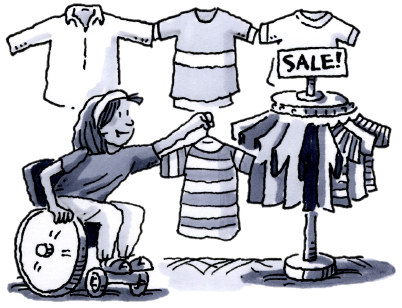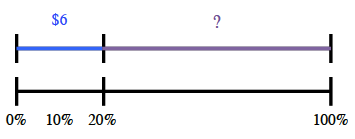### Home > CC1MN > Chapter 11 > Lesson 11.2.3 > Problem11-60

11-60.Calculate the sale prices of each of the following discounted items.

1. A shirt originally priced at $30$ has been discounted $20\%$.

Since the discount is $20\%$, that means that the shirt costs $80\%$ of the original price.
Try making a diagram, like the one below, to help you solve this problem.$24$

2. A sofa originally priced at $1500$ has been discounted $30\%$.

This problem is very similar to part (a).
$10\%$ of $1500$ is $150$.

$1,050$

3. A loaf of bread originally priced at $\3.49$ has been discounted $10\%$.

This problem is very similar to parts (a) and (b).